### Practical Geometry-Solutions 14.2

CBSE Class –VI Mathematics
NCERT Solutions
Chapter 14 Practical Geometry (Ex. 14.2)

Question 1. Draw a line segment of length 7.3 cm, using a ruler.
(i) Place the zero mark of the ruler at a point A.
(ii) Mark a point B at a distance of 7.3 cm from A.
(iii) Join AB.
$\overline{\text{AB}}$ is the required line segment of length 7.3 cm.
(or)
(i) Place one of the pointers of the divider anywhere on the ruler say at 2 cm and count 7.3 cm and open the divider till there, in this case, it is 9.3cm. (It need not be started at 0 mark all the time).
(ii) remove the divider from the ruler and place it on the paper without disturbing and apply a small pressure so that the paper gets a prick from the needles of the divider.
(iii) Mark the pricks as points A and B
(iv) Join the points using a ruler.
Question 2. Construct a line segment of length 5.6 cm using ruler and compasses.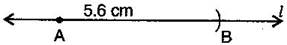(i) Draw a line ${}^{\prime }{l}^{\prime }.$ Mark a point A on this line.
(ii) Place the compasses pointer on zero mark of the ruler. Open it to place the pencil point up to 5.6 cm mark.
(iii) Without changing the opening of the compasses. Place the pointer on A and cut an arc ${}^{\prime }{l}^{\prime }$ at B.
$\overline{\text{AB}}$ is the required line segment of length 5.6 cm.
Question 3. Construct $\overline{\text{AB}}$ of length 7.8 cm. From this cut off $\overline{\text{AC}}$ of length 4.7 cm. Measure $\overline{\text{BC}}.$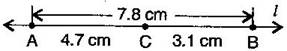(i) Place the zero mark of the ruler at A.
(ii) Mark a point B at a distance 7.8 cm from A.
(iii) Again, mark a point C at a distance 4.7 from A.
By measuring $\overline{\text{BC}}$, we find that BC = 3.1 cm
Question 4. Given $\overline{\text{AB}}$ of length 3.9 cm, construct $\overline{\text{PQ}}$ such that the length $\overline{\text{PQ}}$ is twice that of $\overline{\text{AB}}$. Verify by measurement.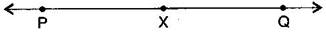(Hint: Construct $\overline{\text{PX}}$ such that length of $\overline{\text{PX}}$ = length of $\overline{\text{AB}}$; then cut off $\overline{\text{XQ}}$ such that $\overline{\text{XQ}}$ also has the length of $\overline{\text{AB}}$.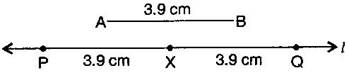(i) Draw a line ${}^{\prime }{l}^{\prime }.$
(ii) Construct $\overline{\text{PX}}$ such that length of $\overline{\text{PX}}$ = length of $\overline{\text{AB}}$
(iii) Then cut of $\overline{\text{XQ}}$ such that $\overline{\text{XQ}}$ also has the length of $\overline{\text{AB}}.$
(iv) Thus the length of $\overline{\text{PX}}$ and the length of $\overline{\text{XQ}}$ added together make twice the length of $\overline{\text{AB}}.$
Verification:
By measurement we find that PQ = 7.8 cm
= 3.9 cm + 3.9 cm = $\overline{\text{AB}}$ + $\overline{\text{AB}}$ = 2 x $\overline{\text{AB}}$
Question 5. Given $\overline{\text{AB}}$ of length 7.3 cm and $\overline{\text{CD}}$ of length 3.4 cm, construct a line segment $\overline{\text{XY}}$ such that the length of $\overline{\text{XY}}$ is equal to the difference between the lengths of $\overline{\text{AB}}$ and $\overline{\text{CD}}.$ Verify by measurement.
(i) Draw a line ${}^{\prime }{l}^{\prime }$ and take a point X on it.
(ii) Construct $\overline{\text{XZ}}$ such that length $\overline{\text{XZ}}$ = length of $\overline{\text{AB}}$ = 7.3 cm
(iii) Then cut off $\overline{\text{ZY}}$ = length of $\overline{\text{CD}}$ = 3.4 cm
(iv) Thus the length of $\overline{\text{XY}}$ = length of $\overline{\text{AB}}$ – length of $\overline{\text{CD}}$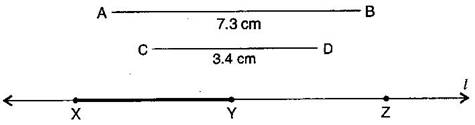By measurement we find that length of $\overline{\text{XY}}$ = 3.9 cm = $\overline{\text{AB}}-\overline{\text{CD}}$ = 7.3 cm – 3.4 cm